# VLOOKUP WildcardArticle byExcelMojo Team## What Is VLOOKUP With Wildcard in Excel?

The VLOOKUP wildcard characters enable one to lookup value using the VLOOKUP() with a partial match. And wildcards work well for numeric and text values.

Users can use the VLOOKUP wildcard characters in scenarios such as looking up the first name in a lookup range of texts containing first and last names. The wildcards in the formula help represent the additional characters missing in the lookup value but present in the value in the lookup range and complete the vertical lookup.

For example, the first table in the image below lists companies, their price change percentages and revenue figures.

If the requirement is to update the revenue data for the companies cited in the second table, using the first table data. And assume the target cells are F3:F4.

Then, we can apply the VLOOKUP wildcard partial match concept in this scenario since the company names given in the second table partially match the names in the lookup range.

Now, look at the above VLOOKUP wildcard partial match example table. We use the VLOOKUP() in the target cells with two wildcard characters, ‘*’ and ‘?’.

We use the wildcard ‘*’ in the lookup_value argument in the cell F3 formula since the company name in cell E3 does not entirely match the values in the given lookup range.

Concatenating the wildcard ‘*’ after the given lookup value tells the function that there are characters following the given lookup value. Then, the VLOOKUP() searches for the value in the lookup range containing the cell E3 value. And this value could have any number of characters after the value in E3.

So, as cell A5 contains the exact match, the VLOOKUP() returns the value in the same row of the column indicated by col_index_num, column C, cell C5, \$284.61 B, as the output.

Meanwhile, we use the wildcard ‘?’ in the target cell F4 VLOOKUP() for the same reason as mentioned earlier. But in this case, the number of characters to ensure the lookup value matches exactly to a value in the lookup range is few.

Concatenating seven question marks and a space character before the given lookup value tells the function that there are eight characters before the given lookup value. Then, the VLOOKUP() searches for the value in the lookup range containing the cell E4 value. And this value must have seven characters and a space character before the value in E4.

So, as cell A6 contains the exact match, the VLOOKUP() returns the value in the same row of the column indicated by col_index_num, column C, cell C6, \$219.50 B, as the output.

###### Key Takeaways
• The VLOOKUP wildcard characters help perform a vertical lookup with a partial match. And the wildcards can represent any character that is not hidden.
• Users can use the VLOOKUP wildcard characters to look up a value containing a part of the value specified in the lookup range in the lookup column.
• We can use two wildcard characters, ‘*’ and ‘?’, in a VLOOKUP function. While the wildcard character ‘*’ helps find a sequence of characters, the ‘?’ represents a single character.
• We must concatenate the wildcard characters with the specified lookup value using the ‘&‘ symbol when using them in the VLOOKUP().

### How To VLOOKUP Wildcards (*, ?) In Excel?

The following VLOOKUP wildcard characters (*, ?) in Excel examples help us avoid VLOOKUP wildcard not working scenarios.

#### #1 – Typical VLOOKUP Function

The steps to use the VLOOKUP() are:

1. Choose the target cell and enter the = VLOOKUP(. [Alternatively, type =V or =VL and double-click the VLOOKUP function from the Excel suggestions]
2. Enter the argument values separated by commas in the VLOOKUP() and close the bracket.
3. Press Enter to execute the VLOOKUP().

[Alternatively, we can choose the target cell and select the Formulas tab → Lookup & ReferenceVLOOKUP function to apply it using the Function Arguments window]

We shall see how a VLOOKUP() behaves when using it without the wildcard characters in a scenario where the lookup value does not have an exact match in the lookup range.

The first table contains an employee list, annual hikes and promotion status.

And the requirement is to update the promotion status for the employees listed in the second table using the data in the first dataset. Assume the target cells are in the range I3:I7.

Then, using the VLOOKUP() in the target cells, with the table_array being an absolute reference to the data range in the first table, will fetch us the required result.

1. Choose the target cell I3, enter the VLOOKUP(), and press Enter.

=VLOOKUP(H3,\$D\$3:\$F\$12,3,0)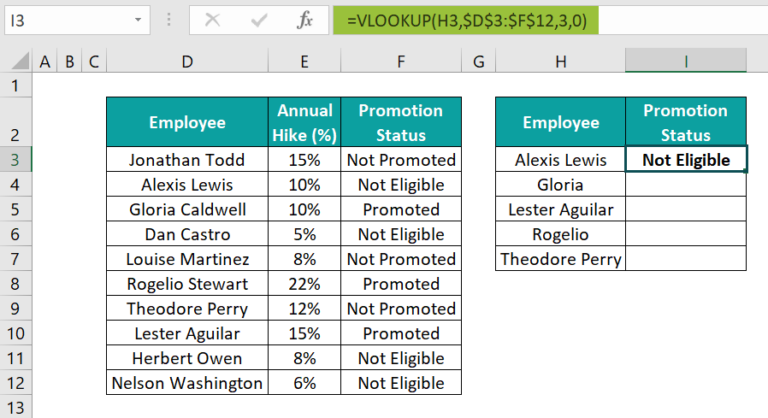[Alternatively, choose cell I3 and FormulasLookup & ReferenceVLOOKUP to open the Function Arguments window.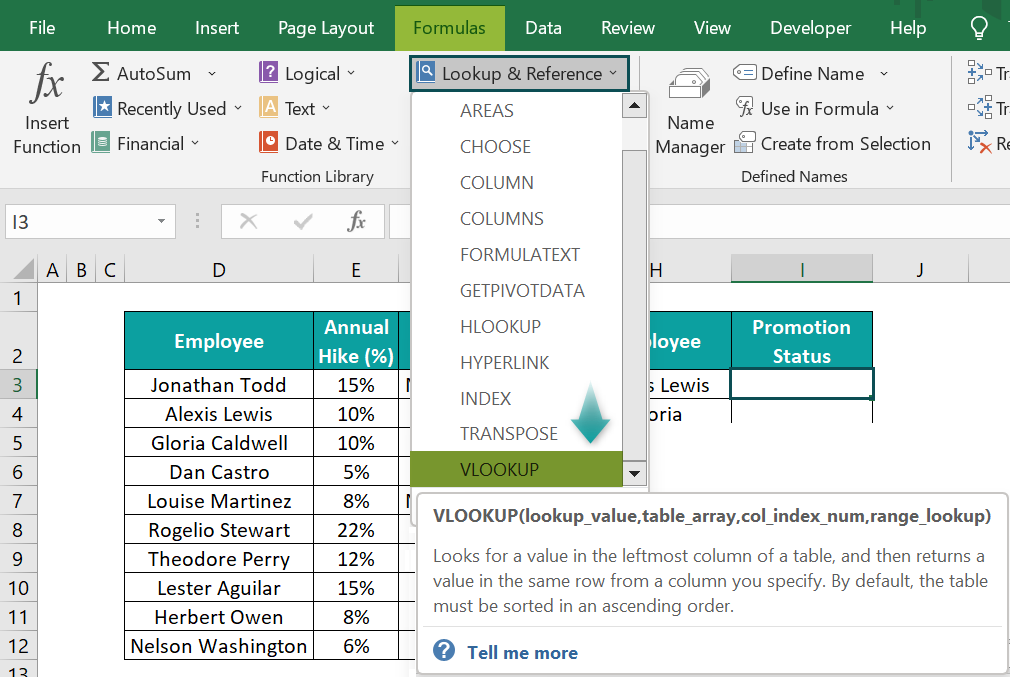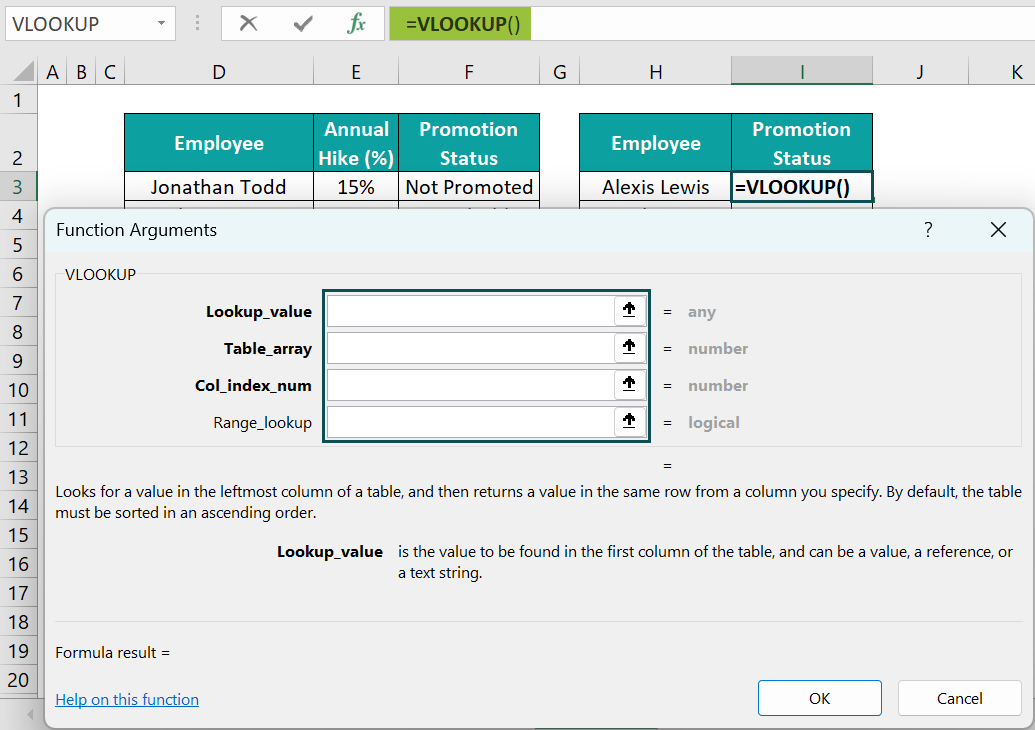Next, update the argument values in the corresponding fields in the Function Arguments window.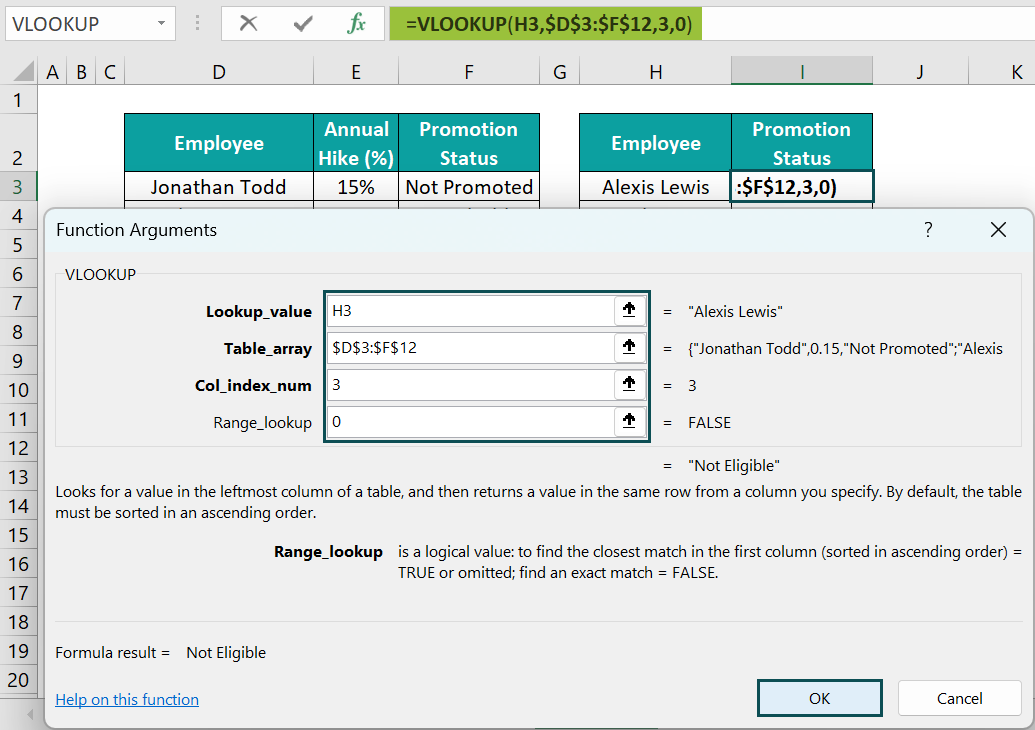Finally, clicking OK will execute the function.]

2. Enter the formula in the remaining target cells using the fill handle.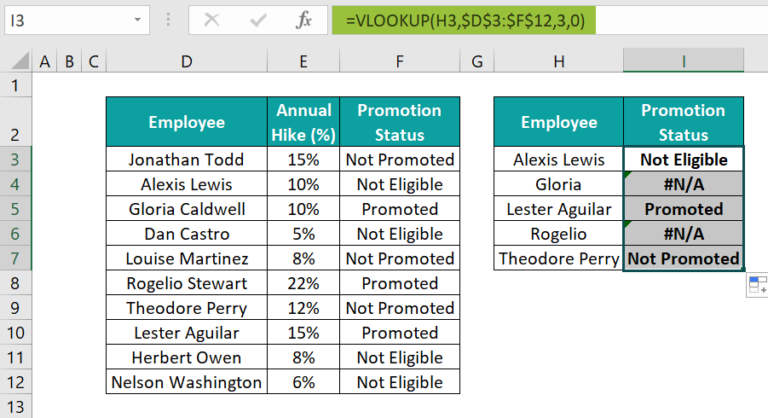Let us see the cell I7 formula to check how it works. The VLOOKUP() accepts the cell H7 value, Theodore Perry, as the lookup value. It looks for the lookup value in the first column in the table_array range, D3:F12, and finds a match in cell D9.

Further, as we must display the promotion status based on the first table data, the col_index_num argument value is 3. Also, we require the function to return a value based on an exact match. So, the last optional argument, range_lookup, will be 0, representing FALSE.

Thus, the function returns the value in row 9 of the third column from the first column in the table_array range, the cell F9 value, Not Promoted, as the outcome.

However, the VLOOKUP() output in the target cells I4 and I6 is the #N/A error.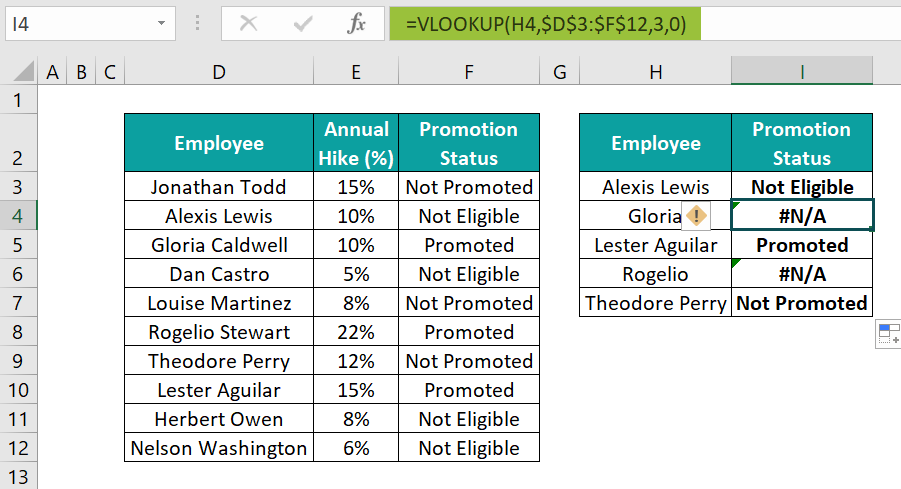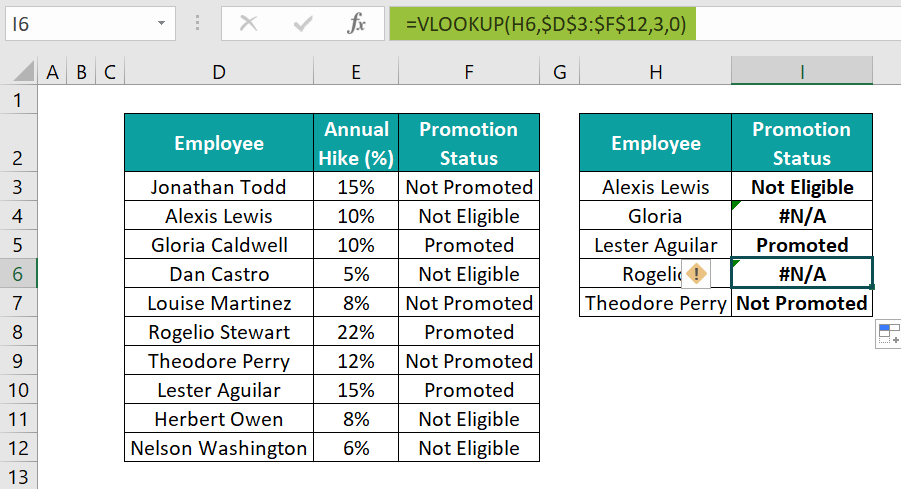The second table does not specify the employees’ full names, as the first column in the specified lookup range. And so, the function fails to find a match for the lookup values, Gloria and Rogelio, while executing in the respective target cells.

In such a case, we can utilize an appropriate VLOOKUP wildcard formula to ensure the function works with partial matching and returns the desired output.

#### #2 – Asterix (*)

The wildcard ‘*’ helps find zero to any number of characters before and after the given lookup value to match it to a value in the specified lookup range.

The steps to use the wildcard character ‘*’ in the VLOOKUP() are:

1. Choose the target cell and enter the = VLOOKUP(.
2. Using the ‘&’ symbol, concatenate the wildcard character ‘*’, within double quotes, before, after (or both) the specified lookup value. The wildcard placement depends on the characters we wish to find with the lookup value in the data.
3. Enter the remaining argument values separated by commas in the VLOOKUP() and close the bracket.
4. Press Enter to execute the VLOOKUP().
##### Example #1

Continuing the previous example, we will use a ‘*’- based VLOOKUP wildcard formula in the target cells to achieve the required outcome.

• Step 1: Choose the target cell I3, enter the VLOOKUP() containing the wildcard ‘*’, and press Enter.

=VLOOKUP(H3&”*”,\$D\$3:\$F\$12,3,0)

• Step 2: Update the formula in the remaining target cells by dragging the fill handle downwards.

The results show the VLOOKUP() works perfectly in all the target cells.

Let us see the cell I4 formula to understand the Excel VLOOKUP wildcard search logic.

The lookup_value argument value is H4&”*”. It implies the function must consider the lookup value as the cell H4 value, Gloria, followed by zero to any number of characters.

Next, the function tries finding a cell in the first column in the lookup range D3:F12, containing the cell H4 value, with additional characters after it. And it locates the match in cell D5.

So, it returns the value in the same row of column F, the cell F5 value, Promoted, as the required employee’s promotion status in the target cell.

##### Example #2

The above-explained formula will work when the lookup range’s first column contains the specified lookup value, followed by additional characters.

On the other hand, assume an employee has a first, middle, and last name mentioned in the lookup column. And the middle name is the specified lookup value in the second table. Then, we may face the possibility of the VLOOKUP wildcard not working in the target cell.

The reason is that the wildcard character ‘*‘ after the specified lookup value in the formula will account for the text following the given lookup value. And we do not have a wildcard character to find the characters before the given lookup value.

For example, consider the first table showing Nobel prize recipients, their category, and the year they won the prize.

And the second table shows some of the recipients’ names. But the names are incomplete and partially match the values in the first column of the first dataset.

If the requirement is to update the year, the recipients listed in the second table won the prize, using the data in the first dataset. Assume the target cells are I3:I8.

Then, here is how to use the ‘*’- based VLOOKUP() in the target cells.

• Step 1: Choose cell I3, enter the VLOOKUP() containing the wildcard character ‘*’, and press Enter.

=VLOOKUP(“*”&H3&”*”,\$C\$3:\$E\$8,3,0)

• Step 2: Drag the excel fill handle till cell I7 to update the formula in all the target cells.

Let us see the cell I7 formula to understand the Excel VLOOKUP wildcard search logic.

The lookup_value argument value is “*”&H7&”*”. It implies the function must consider the lookup value to be the cell H7 value, Luther King, with zero to any number of characters before and after it.

Next, the function tries finding a cell in the first column in the lookup range C3:E8, containing the cell H7 value, with additional characters before and after it. And it locates the match in cell C7.

Thus, it returns the value in the same row of column E, the cell E7 value, 1964, as the required year value in the target cell.

Moreover, the ‘*’ concatenated before and after the cited lookup value finds all the characters before and after the value to find a match in the first column of the table array. So, we can use the above formula, irrespective of what part of the value listed in the first column of the lookup range is provided as the lookup value.

#### #3 – Question Mark (?)

The wildcard character ‘?‘ accounts for one character. Thus, it is useful when we know a few characters precede, succeed, or are anywhere between the cited lookup value.

The steps to use the wildcard character ‘?’ in the VLOOKUP() are:

1. Choose the target cell and enter the = VLOOKUP(.
2. Using the ‘&’ symbol, concatenate the wildcard character ‘?’ within double quotes before, after (or both) the specified lookup value. The wildcard count and placement will depend on the total characters we wish to find with the lookup value in the values provided in the lookup column.
3. Enter the remaining argument values separated by commas in the VLOOKUP() and close the bracket.
4. Press Enter to execute the VLOOKUP().

For example, the first table in the image below lists item codes, items, availability status, costs and order dates.

If the requirement is to update the order dates in column M cells, with the Date data format, for the item codes specified in column K cells of the second table. Then, here is how to use the ‘?’-based VLOOKUP() in the target cells to obtain the required data.

• Step 1: Choose cell M3, enter the VLOOKUP() containing the wildcard character ‘?’, and press Enter.

=VLOOKUP(K3&”-????-??”,\$D\$3:\$H\$8,5,0)

• Step 2: Drag the fill handle to cell M4 to copy the formula into the cell.

Let us check the cell M4 formula to see the formula logic.

The lookup_value argument is K4&”-????-??”. It implies the function must consider the lookup value as the cell K4 value, DELL, followed by a ‘‘, four characters, a ‘‘, and two characters. We use sets of four and two question marks based on the item code format for desktops.

Next, the function tries finding a cell in the first column in the lookup range D3:H8, containing the cell K4 value with the additional characters mentioned above. And it locates the match in cell D8.

Thus, it returns the value in the same row of column H, the cell H8 value, 3/14/2023, as the required order date in the target cell.

• Step 3: Choose cell M5, enter the VLOOKUP() containing the wildcard character ‘?’, and press Enter.

=VLOOKUP(K5&”-????-???”,\$D\$3:\$H\$8,5,0)

Furthermore, the VLOOKUP() logic in cell M5 remains the same as explained earlier. But we use sets of four and three question marks based on the item code format for laptops.

### Important Things To Note

• Use the VLOOKUP wildcardcharacters carefully when the lookup value can match more than one value in the lookup column in the table array. It is because the function returns the first match, and a partial match may give an erroneous result.
• Use the VLOOKUP wildcard characters carefully when the VLOOKUP() must find the required data based on an approximate match, as the match works only with numbers.
• Check the count and position of the ‘?’ wildcard characters included in the VLOOKUP() before executing the function. Because the wildcard represents a single character.

1. Do VLOOKUP wildcards work with IF statements in Excel?

The VLOOKUP wildcards do not work with IF statements in Excel if we use the wildcards directly in the IF().

However, we can use the wildcards inside the VLOOKUP() function. And then, we can apply the wildcard-based VLOOKUP() with the IF() to achieve the desired results.

For example, the first table lists students and their test scores.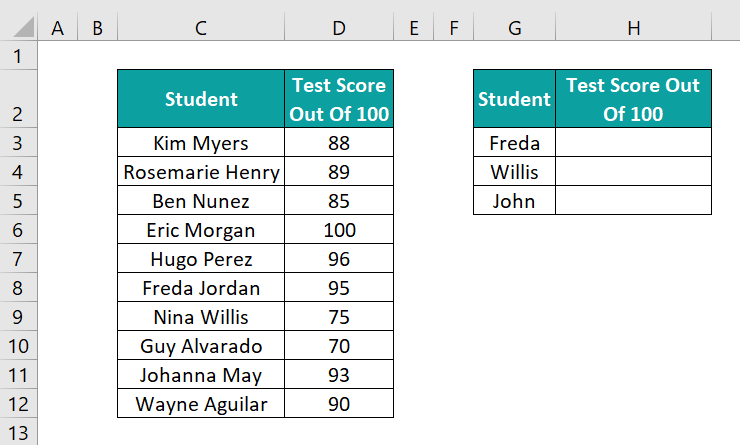Now, assume we have to update the test scores for the students listed in the second table using the first dataset. And we must display the Data Not Found message in the target cell if the data of the specified student is not in the first table. Assume the target cells are H3:H5.

Then, here is how to use the wildcard-based VLOOKUP() in the IF() in the target cells.

• Step 1: Choose cell H3, enter the wildcard-based VLOOKUP() inside the IF(), and press Enter.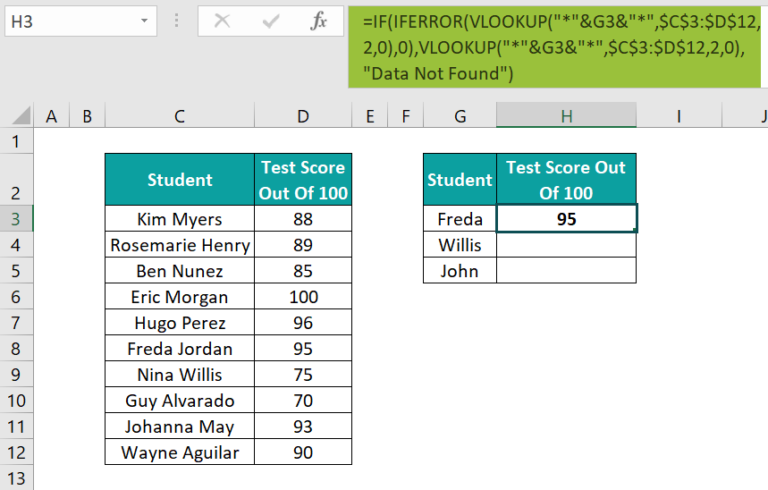Here is how the formula works.

First, the IF() checks the given condition, which is a VLOOKUP() within the IFERROR(). So, the VLOOKUP() accepts the lookup_value argument as “*”&G3&”*”. It indicates that the function must consider the lookup value to be the cell G3 value, Freda, with any number of characters before and after it.

Next, the function tries finding a cell in the first column in the lookup range C3:D12, containing the cell G3 value, with the additional characters preceding and succeeding it. And it locates the match in cell C8.

Thus, it returns the value in the same row of column D, the cell D8 value, 95, as the required test score in the target cell.

Next, the IFERROR() checks if the VLOOKUP() output is an error value. And since it is not, the IFERROR() returns 95 as the output.

Further, as the IF() condition is a non-zero value, 95, the condition holds. And thus, the IF() returns the TRUE value, which is again the same VLOOKUP(), provided as the IF() condition. And thus, the VLOOKUP() returns the value of 95. And it is the IF() output in the target cell.

• Step 2: Drag the fill handle till cell H5 to update the formula in the remaining target cells.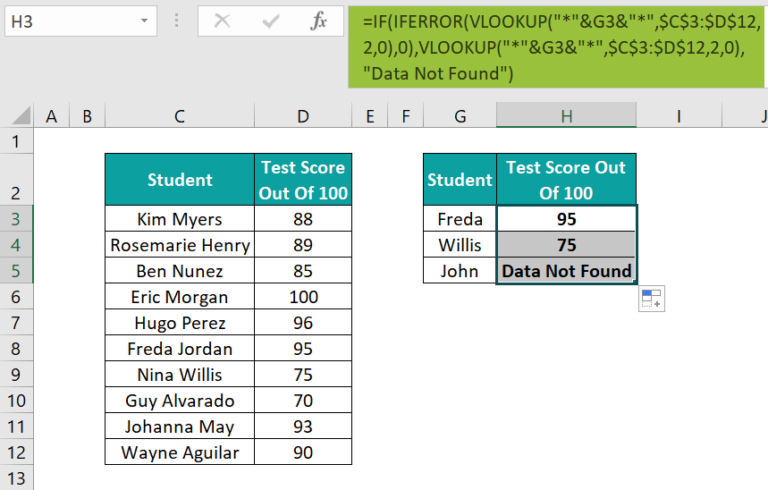We see that the formulas give the desired results in cells H3:H4.

However, in cell H5, the VLOOKUP() output is the #N/A error. And the reason is that, even with the wildcard character, ‘*’, the VLOOKUP() cannot find a match for the lookup value, cited in cell G3, in the lookup column. And hence, the IFERROR() returns 0 in the place of the #N/A error.

Finally, as 0 indicates the IF() condition is FALSE, the function returns the FALSE value, “Data Not Found”, in the target cell.

2. How VLOOKUP wildcard is different from XLOOKUP wildcard?

The VLOOKUP wildcard is different from XLOOKUP wildcard in the following ways:
The VLOOKUP wildcard only looks for values on the right side of the lookup column with a partial match. But the XLOOKUP wildcard searches for values to the left and right of the lookup column with a partial match.
The VLOOKUP wildcard finds the lookup value from the top of the lookup column with a partial match. But the search mode in the XLOOKUP with the wildcard helps decide whether to start finding the lookup value from the top or bottom of the lookup column with a partial match.

3. What are the limitations of using VLOOKUP wildcard?

Typically Excel wildcards can search up to 255 characters, and the VLOOKUP wildcard characters are no exception.
Using VLOOKUP wildcard characters with approximate match (range_lookup argument value of TRUE) can lead to the VLOOKUP() returning an incorrect value. The reason is that the approximate match works only with numeric values.
When the lookup value matches multiple values in the lookup range, the VLOOKUP wildcard characters may lead the function to return an incorrect value. It is because the function returns the first match it finds, which might not be the desired outcome.